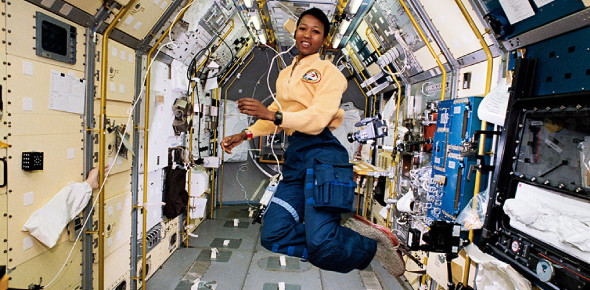# Microgravity Questions: Trivia Quiz!

10 Questions | Total Attempts: 98Settings. ​​​​​​

• 1.
What is Microgravity?
• A.

A really really really really tiny piece of gravity

• B.

An environment where weight is a lot less than what it would be in regular gravity

• C.

Too tiny to know the answer

• D.

No gravity at all.

• 2.
You can simulate microgravity here on earth where we have regular gravity.
• A.

True

• B.

False

• 3.
Newton's first law says:
• A.

Force = Mass X Acceleration

• B.

The cowboys are the greatest football team ever!

• C.

An object in motion stays in motion or at rest until acted on by another force

• D.

For every action there is an equal and opposite reaction

• 4.
Newton's second law says...
• A.

F = Mass X Acceleration

• B.

An object in motion or at rest will stay in motion or at rest, until a force acts on it.

• C.

For every action there is an equal and opposite reaction

• D.

YOU HAVE TO COME TO THE CUBS FIRST FOOTBALL GAME ON THE 29th! GO CUBS!

• 5.
Newton's third law says...
• A.

Force = Mass X Acceleration

• B.

An object at rest will stay at rest until moved by something, and an object in motion will stay in motion until stopped by something.

• C.

If a tree falls in the forest and nobody is around, then it didn't make a sound anyway.

• D.

For every action, there is an equal and opposite reaction

• 6.
What is the meaning of the word "Orbit"
• A.

The curved path of an object or spacecraft around a star, planet, or moon

• B.

To float near an object in space

• C.

To be pulled around by the gravity of an object

• D.

To get an attitude with someone you really don't like

• 7.
What does "Freefall" mean in this class?
• A.

What my grade will do if I don't pay attention.

• B.

Letting someone get some some of your drink without touching their mouth on it.

• C.

Jumping off of something without having to pay for it.

• D.

Falling in a gravitational field where the acceleration is the same as that due to gravity alone.

• 8.
The acceleration of Earth's gravity is...
• A.

Faster than on Mars

• B.

9.8 m^2

• C.

28 Mph

• D.

I dunno... Fast?

• 9.
Gravitation is the attraction of objects due to their masses
• A.

True

• B.

False

• 10.
YOU create an attractive force in our universe?
• A.

True

• B.

False

Related TopicsBack to top